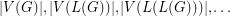# Graham's conjecture on tree reconstruction

 Importance: Medium ✭✭
 Author(s): Graham, Ronald L.
 Subject: Graph Theory » Basic Graph Theory
 Keywords: reconstruction tree
 Posted by: mdevos on: March 18th, 2007
Problem   for every graph, we letdenote the line graph of. Given thatis a tree, can we determine it from the integer sequence?

Graph reconstruction is a notoriously difficult subject. This conjecture is an unusual type of reconstruction problem where our class of graphs is very limited - just trees, but we are also given relatively little information - just a sequence of integers.

## Bibliography

[GR] C. Godsil and G. Royle, Algebraic graph theory. Graduate Texts in Mathematics, 207. Springer-Verlag, New York, 2001 (page 18).

* indicates original appearance(s) of problem.

### Reference

Could someone pleas give a proper reference? If I'm not mistaken, the problem is just *mentioned* in G&R, without references (anyway, I didn't find any).

### Graph theory

Does for any tree T there exist n that L^n(T) is a regular graph? Or perhaps for all graph?

### No

Consider L^i(T) is a graph with "even triangle"(triangle with even degrees of vertices) subgraph. Edges of even triangle produce new even triangle in L^(i+1)(T). And if there is an odd degree vertex adjacent to parent triangle, there would be another one adjacent to child. So, irregular subgraph remains.

### No

Let G be a star graph of order 5. Then L(G) = C_4. Note that L(C_4) = C_4.

### If G is a star graph of

If G is a star graph of order 5, then L(G) = K_5.